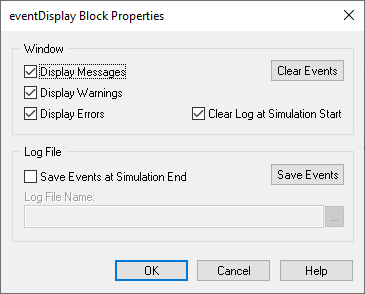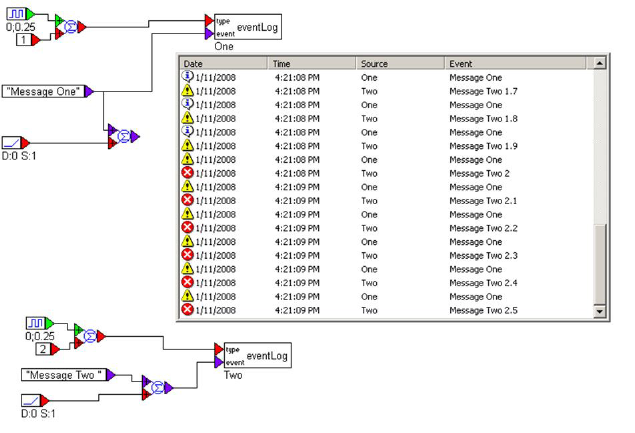## /Block Category: Arithmetic

Inputs: Real or complex scalars, vectors, or matrices.

l: numerator x1.

r: denominator x2. If r = 0 or is unconnected, Embed displays a “Divide by 0” message and highlights the offending block in red.

Description: The / block generates the quotient of the input signals. To perform matrix inversions, use the invert block.Label: Indicates a user-defined block label that appears when View > Block Labels is activated.

#### Examples

1. Division of two scalar inputs

Consider the equation y = 24/32 realized as:2. Division of a vector by a scalar

Consider the equation:

y = x/24

where x = [12 24 36] realized as:When the simulation runs, the / block divides each element of vector x with the constant value of 24.

3. Division of vectors

Consider the equation:

w = x/y

where x = [12 24 36] and y = [6 12 18] realized as:When the simulation runs, the / block performs an element-by-element division operation on the incoming vectors. For example, w(1) = x(1)/y(1), w(2) = x(2)/y(2), and so on.